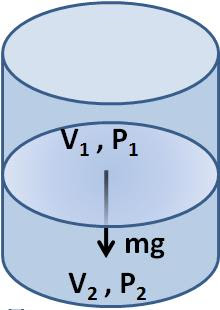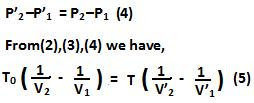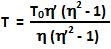## Tuesday, January 19, 2010

### Irodov Problem 2.6Let the initial pressure and volume of the upper partition be P1, V1 and that of the lower partition be P2,V2. We know that each of the partitions has 1 mole of gas. Hence, from Ideal gas law equation we have,What (2) shows is that there is a pressure difference between the lower and upper partitions initially. It is not clear as to why this is so from the problem. A plausible cause might be that the partitioning wall might have a weight, say mg. Then (P2-P1)A = mg, where A is the surface area of the partitioning wall. This is indicated in the figure.

Now the temperature of the system is changed to a new temperature say T. Then let the corresponding volumes and pressures of the partitions be P'1,V'1,P'2,V'2. Similar to Eqn (2) we can derive,But then the pressure difference has to be the same since, the weight of the partition has not changed. Hence we have,We know however that,From (6a-d) we have,From (7a-d) and (5) we obtain,1.1.